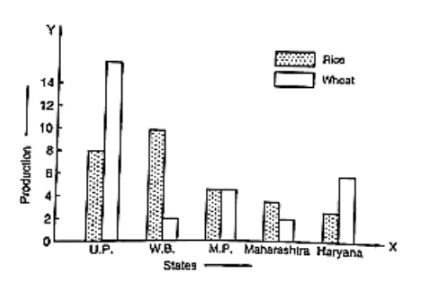Question:(i) What information is given by the bar graph?

(ii) Which state is the largest producer of rice?

(iii) Which state is the largest producer of wheat?

(iv) Which state has total production of rice and wheat at its maximum?

(v) Which state has total production of wheat and rice at its minimum?

Solution:

(i) The bar graph represents the production of rice and wheat in different states of India.

(ii) According to the height of the bars corresponding to rice, W.B. is the largest producer of rice.

(iii) According to the height of the bars corresponding to wheat. U.P. is the largest producer of wheat.

(iv) U.P. has the maximum total production of rice and wheat, which is 8 + 16 = 24 units

(v) Maharashtra has the minimum total production of rice and wheat, which are exactly 2 + 4 = 6 units.